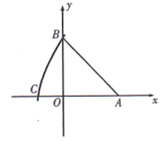$\text{A.}$ $(3 \sqrt{2}, 0)$ $\text{B.}$ $(\sqrt{2}, 0)$ $\text{C.}$ $(-\sqrt{2}, 0)$ $\text{D.}$ $(-3 \sqrt{2}, 0)$
【答案】 C